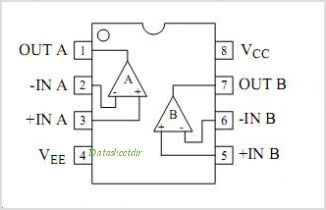# -=SOLVED=- Thermistor

Is the termistor supposed to get really hot? Or is my wiring screwed up?

it should be embient temperature.

Did you have a picture of your termistor's wiring?

Being a bit of a grammar nazi here, but in the interest of helping non-english speakers:

thermister, ambientBTW - your thermistor should -not- be heating up - as liudr noted, a pic or schematic would help...

Thanks cr0sh. There exist applications of heating up thermistors with large current and we couldn't tell until the OP shares more info. If you want to tell liquid level, you can use the liquid as heat sink and apply larger current on the thermistors so only those ones in the liquid will stay cool and have large resistance. The ones outside liquid are hot and less resistive.

It looks like its the amplifier that gives out the heat and not the thermistor it self. bought it from ebay.
http://cgi.ebay.com/Analog-Temperature-Sensor-Module-Arduino-Shield-/270669450328

Im using the The Elaborate Code from the playground.
http://www.arduino.cc/playground/ComponentLib/Thermistor2

The sensor is giving 60deg C at about 20deg C?

It looks like its the amplifier that gives out the heat and not the thermistor it self. bought it from ebay.

That's not good either. It looks like a simple opamp and no way should it be getting hot. Are you sure you wired it correctly and not reversed the power and ground leads?

Lefty

Probably think you are right about the wiring. As seen on the picture the wiring described on the board does not work at all. Even my arduino board lost connection to my computer wired like that. turning the pins the other way around gives me 60deg C. Adding heat or cold gives me minor differences on the reading. Have tryed all 6 combos on the wiring with no luck at all. ( probably fried the whole thing.)

Im not so familiar with electronics so maybe this will give you an idea how its supposed to be connected.

here is my sensor

heres the amplifier on the board.Karl

From the picture, top pin is signal, middle is 5V and bottom is ground. How did you wire it when it reads 60DegC?

it shows 60deg C as wired oposite on the picture. amlifier gets super hot when i do this.

changing the acc and ground give me -11 and normal temp on amplifier.

As mentioned earlyer, connecting it like on the picture make the arduino loose connection with the pc. and the amplifier gets super hot.

have no idea whats wrong. its shurely the wiring

Well, maybe your code is wrong reporting -11DegC. Care to share your code for a diagnostic?

Posted by: liudr
Well, maybe your code is wrong reporting -11DegC. Care to share your code for a diagnostic?

I think you are correct. Thats the only thing that makes sense to me. The thermistor gives steady signals that behave normal when exposed too heat or cold.

I have fiddled around with two codes from the playground.

The easy one

``````#include <math.h>

double Temp;
Temp = 1 / (0.001129148 + (0.000234125 + (0.0000000876741 * Temp * Temp ))* Temp );
Temp = Temp - 273.15;            // Convert Kelvin to Celcius
Temp = (Temp * 9.0)/ 5.0 + 32.0; // Convert Celcius to Fahrenheit
return Temp;
}

void setup() {
Serial.begin(115200);
}

void loop() {
delay(100);
}
``````

The Elaborate Code

``````#include <math.h>

#define ThermistorPIN 0                 // Analog Pin 0

float vcc = 4.91;                       // only used for display purposes, if used
// set to the measured Vcc.
float thermr = 10000;                   // thermistor nominal resistance

long Resistance;
float Temp;  // Dual-Purpose variable to save space.

Temp = log(Resistance); // Saving the Log(resistance) so not to calculate  it 4 times later
Temp = 1 / (0.001129148 + (0.000234125 * Temp) + (0.0000000876741 * Temp * Temp * Temp));
Temp = Temp - 273.15;  // Convert Kelvin to Celsius

// BEGIN- Remove these lines for the function not to display anything
//Serial.print("/1024");                           // Print out RAW ADC Number
//Serial.print(", vcc: ");
//Serial.print(vcc,2);
//Serial.print(" Kohms, Volts: ");
//Serial.print(", Resistance: ");
//Serial.print(Resistance);
//Serial.print(" ohms, ");
// END- Remove these lines for the function not to display anything

// Uncomment this line for the function to return Fahrenheit instead.
//temp = (Temp * 9.0)/ 5.0 + 32.0;                  // Convert to Fahrenheit
return Temp;                                      // Return the Temperature
}

void setup() {
Serial.begin(115200);
}

void loop() {
float temp;
Serial.print("Celsius: ");
Serial.print(temp,1);                             // display Celsius
//temp = (temp * 9.0)/ 5.0 + 32.0;                  // converts to  Fahrenheit
//Serial.print(", Fahrenheit: ");
//Serial.print(temp,1);                             // display  Fahrenheit
Serial.println("");
delay(5000);                                      // Delay a bit...
}
``````

That code is not intended for generic use, I believe it is intended for use with 10k thermistors.

This code is the steinhart-hart equation:

``````Temp = 1 / (0.001129148 + (0.000234125 * Temp) + (0.0000000876741 * Temp * Temp * Temp));
``````

Which converts the analog reading to "Kelvins". The line underneath that then converts Kelvins to Celsius.

For your thermistor to work correctly, it will need to be calibrated. That is, you need to calculate your own 3 constants (the numeric values) for the equation to work for your thermistor.

Wiring was messed up. Calibration using math solved the issue!
Thanks for all of the help i got!